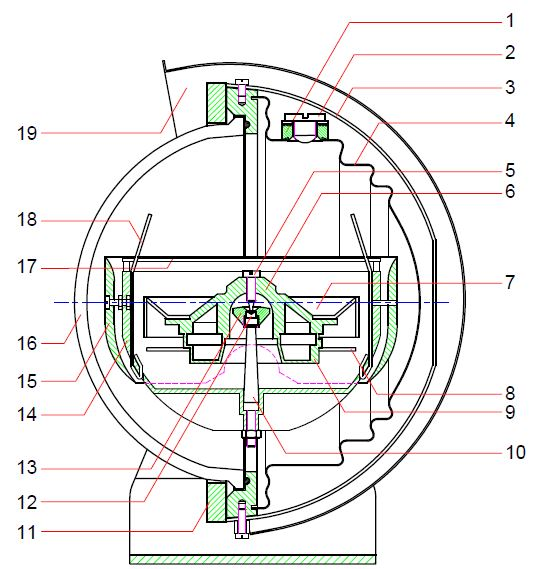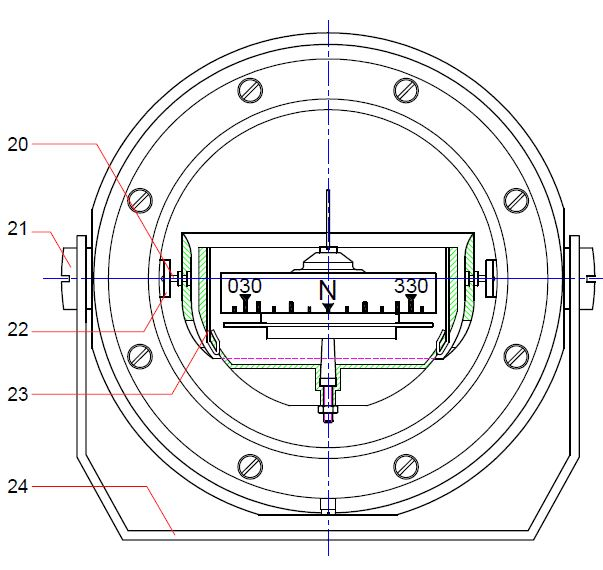# Construction of a spherical compass

The following describes the construction of a magnetic compass using our IOTA/2 model.
This is a spherical compass whose construction is similar to our other spherical compass models.1 = Filler hole 2 = Filling screw 3 = Protective cover 4 = Diaphragm 5 = Pivot 6 = Floater top side 7 = Compass card 8 = Damper 9 = Floater bottom side 10 = Jewel column 11 = Cover ring 12 = Jewel 13 = Jewel socket14 = Gimbal system inner part 15 = Gimbal system outer part 16 = Glas dome 17 = Damping disk 18 = Long lubber line 19 = Hinged protective cover 20 = Inner axis 21 = Outer axis 22 = Angle 23 = Short lubber line 24 = Bracket